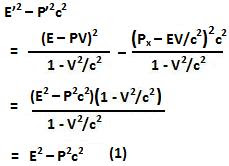## Friday, January 8, 2010

### Irodov Problem 1.383

We can use the results from Problem 1.381 to prove this invariance result.What Eqn (1) is indicates is an invariance since the quantity does not depend which inertial frame you are observing from!

The value of the constant can be determined simply by considering a frame that is moving with the particle itself. In such a frame, the particle is absolutely stationary, in other words P=0. The energy is equal to the rest mass energy and so we have,